# Painting a hut

It is necessary to paint the exterior walls of hut whose layout is a rectangle of 6.16 m x 8.78 m wall height is 2.85 meters. Cottage has five rectangular windows; three have dimensions of 1.15 m x 1.32 m and two 0,45 m x 0.96 m. How many m2 is necessary to paint?

S =  79.74 m2

### Step-by-step explanation: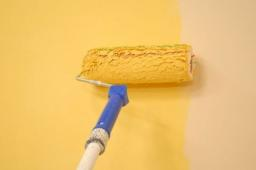Did you find an error or inaccuracy? Feel free to write us. Thank you!Tips to related online calculators
Do you want to convert length units?

#### You need to know the following knowledge to solve this word math problem:

We encourage you to watch this tutorial video on this math problem:

## Related math problems and questions:

• Jared's room paintingJared wants to paint his room. The room's dimensions are 12 feet by 15 feet, and the walls are 9 feet high. Two windows measure 6 feet by 5 feet each. There are two doors, whose dimensions are 30 inches by 6 feet each. If a gallon of paint covers approxim
• PaintingTo paint the pool with dimensions: 2 meters depth, 3m x 4m we bought paint to 50 meters square. How many "paint" will be a waste?
• Wall painting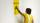The wall is 4 meters wide and 2 meters high. The window in the wall has dimensions of 2x1,8 meters. How many liters of color is needed to paint two-layer these walls, if the 1 m2 needs 1 liters of paint?
• Insulate houseThe property owner wants to insulate his house. The house has these dimensions 12, and 12 m is 15 m high. The windows have 6 with dimensions 170 and 150 cm. Entrance doors are 250 and 170 cm in size. How many square meters of polystyrene does he need?
• PaintingThe room is long 50 meters and wide 60dm and 300 cm high. Calculate how much it will cost painting if area of windows and doors is 15% of the total area. One square meter cost 50cents.
• The wall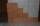The wall in the bathroom has dimensions of 2.8 m and 2.6 m. If we would like to cover this wall to a height of 1.6 m. How many square meters of tiles do we need to buy?
• Room dimensions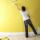Room dimensions are 5m and 3.5m. Room height is 2.85m. Paint the room (even with the ceiling). There will be 2 layers. Doors and windows have a total of 2.5 m2. One box of paint is enough for 6m2. How many boxes of paint are needed? How much do we pay if
• If one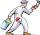If one liter of pants covers an area of 5 m2, how much paint is needed to cover: a) rectangular swimming pool With dimensions 4m x 3m x 2.5m (the Inside walls and the floor only) b) the Inside walls and floor of a cylindrical reservoir with a diameter of
• The roomThe room has a cuboid shape with dimensions: length 50m and width 60dm and height 300cm. Calculate how much this room will cost paint (a floor is not painted) if the window and door area is 15% of the total area and 1m2 cost 15 euro.
• Water levelHow high is the water in the swimming pool with dimensions of 37m in length and 15m in width, if an inlet valve is opened for 10 hours flowing 12 liters of water per second?
• Bricks wallThere are 5000 bricks. How high wall thickness of 20 cm around the area which has dimensions 20 m and 15 m can use these bricks to build? Brick dimensions are 30 cm, 20 cm and 10 cm.
• PoolThe prism-shaped pool is 2 m deep with a bottom of the isosceles trapezoid with base dimensions of 10 m and 18 m and arm legs 7 m long and 5.7 m long. During the spring cleaning, the bottom and walls of the pool must be painted. How many m2 of paint shoul
• Cellar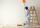Cellar for storing fruit has a rectangular base with sides 14 m and 7 meters. You should paint sidewall to 2 m. How m2 surface must be painted?
• Surface and volume od cuboidContent area of the square base of cuboid is Sp = 36 cm2 and its height 80 mm. Determine its surface area and volume.Calculate the surface of a quadrilateral prism according to the input: Area of the diamond base S1 = 2.8 m2, length of the base edge a = 14 dm, height of the prism 1,500 mm.a) Dusan break two same window, which has triangular shape with a length of 0.8 m and corresponding height 9.5 dm. Find how many dm2 of glass he needs to buy for glazing of these windows. b) Since the money to fix Dusan has not, must go to the paint jobCalculate the surface of a regular triangular prism with a bottom edge of 8.5 meters and an appropriate height of 60 meters, and prism height is 1.4 meters.